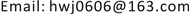﻿ 一类三阶非线性微分方程两点边值问题的变号解 Sign-Changing Solutions for Two-Point Boundary Value Problems of Three-Order Nonlinear Differential Equations

Vol.06 No.08(2017), Article ID:22805,7 pages
10.12677/AAM.2017.68116

Sign-Changing Solutions for Two-Point Boundary Value Problems of Three-Order Nonlinear Differential Equations

Hongwei Ji

Department of Mathematics and Physics, Nantong Normal College, Nantong JiangsuReceived: Oct. 11th, 2017; accepted: Nov. 1st, 2017; published: Nov. 27th, 2017ABSTRACT

In this paper, we use the fixed point theorem with Banach lattice structure and topological degree to discuss the three-order two-point boundary value problem $-{u}^{‴}\left(t\right)=f\left(u\left(t\right)\right)$ for all $t\in \left[0,1\right]$ subject to $u\left(0\right)={u}^{\prime }\left(0\right)={u}^{″}\left(1\right)=0$ , where $f\in C\left(R,R\right)$ . If $f$ satisfies certain conditions, then the existence result of the sign-changing solution is obtained. Moreover, if $f$ is odd for all $t\in \left[0,1\right]$ , then the problem has two sign-changing solutions.

Keywords:Lattice Structure, Third-Order Two-Point Boundary Value Problem, Sign-Changing Solutions1. 引言

$\left\{\begin{array}{l}-{u}^{‴}\left(t\right)=f\left(u\left(t\right)\right),t\in \left[0,1\right]\\ u\left(0\right)={u}^{\prime }\left(0\right)={u}^{″}\left(1\right)=0\end{array}$ (1)

(H1) $f\left(0\right)=0$$f\left(u\right)$ 关于 $u$ 严格递增；

(H2) $\underset{|u|\to +\infty }{\mathrm{lim}}\frac{f\left(u\right)}{u}={\beta }_{1}$ 关于 $t$$\left[0,1\right]$ 上一致，且存在正整数 ${n}_{1}$ 使得 ${\lambda }_{2{n}_{1}}<{\beta }_{1}<{\lambda }_{2{n}_{1}+1}$ ；其中， $0<{\lambda }_{1}<{\lambda }_{2}<\cdots <{\lambda }_{n}<{\lambda }_{n+1}<\cdots$ 是方程 ${\text{e}}^{-\frac{3}{2}\sqrt{\lambda }}+2\mathrm{cos}\frac{\sqrt{3}}{2}\sqrt{\lambda }=0$ 的无穷多个正解；

(H3) $\underset{u\to 0}{\mathrm{lim}}\frac{f\left(u\right)}{u}={\beta }_{0}$ 关于 $t$$\left[0,1\right]$ 一致成立，且 $0<{\beta }_{0}<{\lambda }_{1}$

2. 预备知识

$P$ 是Banach空间 $E$ 中的锥， $E$ 中的半序由锥 $P$ 导出。若存在常数 $N>0$ ，使得 $\theta \le x\le y⇒‖x‖\le N‖y‖$ ，则称 $P$ 是正规锥。如果 $P$ 含有内点，即 $P$ 的内部 $\mathrm{int}P\ne \varnothing$ ，则称 $P$ 是体锥。

$E$ 在半序 $\le$ 下成为一个格，即对任意的 $x,y\in E$$\mathrm{sup}\left\{x,y\right\}$$\mathrm{inf}\left\{x,y\right\}$ 都存在。对 $x\in E$${x}^{+}=\mathrm{sup}\left\{x,\theta \right\}$${x}^{-}=\mathrm{sup}\left\{-x,\theta \right\}$ ，分别称为 $x$ 的正部和负部， $|x|={x}^{+}+{x}^{-}$ 称为 $x$ 的模。显然， ${x}^{+}\in P$${x}^{-}\in \left(-P\right)$$|x|\in P$$x={x}^{+}-{x}^{-}$

$E=C\left[0,1\right]$ ，范数 $‖u‖={\mathrm{max}}_{t\in \left[0,1\right]}|u\left(t\right)|$$P=\left\{u\in E:u\left(t\right)\ge 0,t\in \left[0,1\right]\right\}$ 。显然 $E$ 是Banach空间。 $P$$E$ 的一个正规体锥，且 $E$ 在锥 $P$ 导出的半序“ $\le$ ”下成为一个格。

$Ku\left(t\right)={\int }_{0}^{1}G\left(t,s\right)u\left(s\right)\text{d}s,t\in \left[0,1\right],\forall u\in E$

$Fu\left(t\right)=f\left(u\left(t\right)\right),t\in \left[0,1\right],\forall u\in E$

$A=KF$

$G\left(t,s\right)=\left\{\begin{array}{l}s\left(t-\frac{s}{2}\right),0\le s\le t\le 1,\\ \frac{1}{2}{t}^{2},0\le t\le s\le 1\end{array}$

$\frac{1}{{\lambda }_{1}}>\frac{1}{{\lambda }_{2}}>\cdots >\frac{1}{{\lambda }_{n}}>\frac{1}{{\lambda }_{n+1}}>\cdots >0$ 分别为线性算子 $K$ 的特征值，且每个特征值 $\frac{1}{{\lambda }_{i}}\left(i=1,2,\cdots \right)$ 的代数重数为1。

$\left\{\begin{array}{l}-{u}^{‴}\left(t\right)=\lambda u\left(t\right),t\in \left[0,1\right]\\ u\left(0\right)={u}^{\prime }\left(0\right)={u}^{″}\left(1\right)=0\end{array}$ (2)

$\lambda >0$ 时，记 $\sqrt{\lambda }=a$ ，则(2)的通解为

$u\left(t\right)={C}_{1}{\text{e}}^{-at}+{C}_{2}{\text{e}}^{\frac{1}{2}at}\mathrm{cos}\frac{\sqrt{3}}{2}at+{C}_{3}{\text{e}}^{\frac{1}{2}at}\mathrm{sin}\frac{\sqrt{3}}{2}at,\text{\hspace{0.17em}}t\in \left[0,1\right]$

$u\left(t\right)={C}_{1}\left({\text{e}}^{-at}-{\text{e}}^{\frac{1}{2}at}\mathrm{cos}\frac{\sqrt{3}}{2}at+\sqrt{3}{\text{e}}^{\frac{1}{2}at}\mathrm{sin}\frac{\sqrt{3}}{2}at\right),\text{\hspace{0.17em}}\text{\hspace{0.17em}}t\in \left[0,1\right]$

${\text{e}}^{-\frac{3}{2}\sqrt{\lambda }}+2\mathrm{cos}\frac{\sqrt{3}}{2}\sqrt{\lambda }=0$ 有无穷多个正解 $0<{\lambda }_{1}<{\lambda }_{2}<\cdots <{\lambda }_{n}<{\lambda }_{n+1}<\cdots$ ，其中 ${\lambda }_{n}={a}_{n}^{3}$$n=1,2,\cdots$ ，所以问

$\mu$ 是线性算子 $K$ 的特征值， $u\in E\\left\{0\right\}$ 是对应的特征函数。则

$\left\{\begin{array}{l}-{u}^{‴}\left(t\right)=\frac{1}{\mu }u\left(t\right),t\in \left[0,1\right]\\ u\left(0\right)={u}^{\prime }\left(0\right)={u}^{″}\left(1\right)=0\end{array}$

$\sqrt{\frac{1}{{\mu }_{n}}}=\sqrt{{\lambda }_{n}}=a$ ，则对应于特征值 $\frac{1}{{\lambda }_{n}}$ 的特征函数为

$u\left(t\right)=C\left({\text{e}}^{-at}-{\text{e}}^{\frac{1}{2}at}\mathrm{cos}\frac{\sqrt{3}}{2}at+\sqrt{3}{\text{e}}^{\frac{1}{2}at}\mathrm{sin}\frac{\sqrt{3}}{2}at\right),\text{\hspace{0.17em}}t\in \left[0,1\right]$

$\mathrm{dim}\mathrm{ker}\left(\frac{1}{{\lambda }_{n}}I-K\right)=\mathrm{dim}\mathrm{ker}\left(I-{\lambda }_{n}K\right)=1$ (3)

$\mathrm{ker}\left(I-{\lambda }_{n}K\right)=\mathrm{ker}{\left(I-{\lambda }_{n}K\right)}^{2}$ (4)

$u\in \mathrm{ker}{\left(I-{\lambda }_{n}K\right)}^{2}$ ，如果 $\left(I-{\lambda }_{n}K\right)u\ne \theta$ ，那么 $\left(I-{\lambda }_{n}K\right)u$ 是线性算子 $K$ 对应于特征值 $\frac{1}{{\lambda }_{n}}$ 的特征函

$\left(I-{\lambda }_{n}K\right)u=\gamma \left({\text{e}}^{-at}-{\text{e}}^{\frac{1}{2}at}\mathrm{cos}\frac{\sqrt{3}}{2}at+\sqrt{3}{\text{e}}^{\frac{1}{2}at}\mathrm{sin}\frac{\sqrt{3}}{2}at\right),\text{\hspace{0.17em}}t\in \left[0,1\right]$

$\left\{\begin{array}{l}{u}^{‴}\left(t\right)+{a}^{3}u\left(t\right)=\gamma \left(-{a}^{3}{\text{e}}^{-at}+{a}^{3}{\text{e}}^{\frac{1}{2}at}\mathrm{cos}\frac{\sqrt{3}}{2}at-{a}^{3}\sqrt{3}{\text{e}}^{\frac{1}{2}at}\mathrm{sin}\frac{\sqrt{3}}{2}at\right),t\in \left[0,1\right]\\ u\left(0\right)={u}^{\prime }\left(0\right)={u}^{″}\left(1\right)=0\end{array}$

$\begin{array}{c}u\left(t\right)={C}_{1}{\text{e}}^{-at}+{C}_{2}{\text{e}}^{\frac{1}{2}at}\mathrm{cos}\frac{\sqrt{3}}{2}at+{C}_{3}{\text{e}}^{\frac{1}{2}at}\mathrm{sin}\frac{\sqrt{3}}{2}at\\ \text{\hspace{0.17em}}\text{\hspace{0.17em}}-\frac{1}{3}\gamma at{\text{e}}^{-at}+\frac{1}{3}\gamma at{\text{e}}^{\frac{1}{2}at}\mathrm{cos}\frac{\sqrt{3}}{2}at+\frac{\sqrt{3}}{3}\gamma at{\text{e}}^{\frac{1}{2}at}\mathrm{sin}\frac{\sqrt{3}}{2}at\end{array}$

${C}_{2}=-{C}_{1},{C}_{3}=\sqrt{3}{C}_{1}$ ，从而

$\begin{array}{c}u\left(t\right)={C}_{1}\left({\text{e}}^{-at}-{\text{e}}^{\frac{1}{2}at}\mathrm{cos}\frac{\sqrt{3}}{2}at+\sqrt{3}{\text{e}}^{\frac{1}{2}at}\mathrm{sin}\frac{\sqrt{3}}{2}at\right)\\ \text{\hspace{0.17em}}\text{\hspace{0.17em}}-\frac{1}{3}\gamma at{\text{e}}^{-at}+\frac{1}{3}\gamma at{\text{e}}^{\frac{1}{2}at}\mathrm{cos}\frac{\sqrt{3}}{2}at+\frac{\sqrt{3}}{3}\gamma at{\text{e}}^{\frac{1}{2}at}\mathrm{sin}\frac{\sqrt{3}}{2}at\end{array}$

$\begin{array}{l}{u}^{″}\left(1\right)={C}_{1}\left({a}^{2}{\text{e}}^{-a}+\frac{1}{2}{a}^{2}{\text{e}}^{\frac{1}{2}a}\mathrm{cos}\frac{\sqrt{3}}{2}a+\frac{3}{2}{a}^{2}{\text{e}}^{\frac{1}{2}a}\mathrm{cos}\frac{\sqrt{3}}{2}a\right)-\frac{1}{3}\gamma a\left(-2a{\text{e}}^{-a}+{a}^{2}{\text{e}}^{-a}\right)\\ \text{\hspace{0.17em}}\text{\hspace{0.17em}}\text{\hspace{0.17em}}\text{\hspace{0.17em}}\text{\hspace{0.17em}}\text{\hspace{0.17em}}\text{\hspace{0.17em}}\text{\hspace{0.17em}}\text{\hspace{0.17em}}\text{\hspace{0.17em}}+\frac{1}{3}\gamma a\left(a{\text{e}}^{\frac{1}{2}a}\mathrm{cos}\frac{\sqrt{3}}{2}a-\sqrt{3}a{\text{e}}^{\frac{1}{2}a}\mathrm{sin}\frac{\sqrt{3}}{2}a-\frac{1}{2}{a}^{2}{\text{e}}^{\frac{1}{2}a}\mathrm{cos}\frac{\sqrt{3}}{2}a-\frac{\sqrt{3}}{2}{a}^{2}{\text{e}}^{\frac{1}{2}a}\mathrm{cos}\frac{\sqrt{3}}{2}a\right)\\ \text{\hspace{0.17em}}\text{\hspace{0.17em}}\text{\hspace{0.17em}}\text{\hspace{0.17em}}\text{\hspace{0.17em}}\text{\hspace{0.17em}}\text{\hspace{0.17em}}\text{\hspace{0.17em}}\text{\hspace{0.17em}}\text{\hspace{0.17em}}+\frac{\sqrt{3}}{3}\gamma a\left(a{\text{e}}^{\frac{1}{2}a}\mathrm{sin}\frac{\sqrt{3}}{2}a+\sqrt{3}a{\text{e}}^{\frac{1}{2}a}\mathrm{cos}\frac{\sqrt{3}}{2}a-\frac{1}{2}{a}^{2}{\text{e}}^{\frac{1}{2}a}\mathrm{sin}\frac{\sqrt{3}}{2}a+\frac{\sqrt{3}}{2}{a}^{2}{\text{e}}^{\frac{1}{2}a}\mathrm{cos}\frac{\sqrt{3}}{2}a\right)=0\end{array}$

${\left(\mathrm{sin}\frac{\sqrt{3}}{2}a\right)}^{2}+{\left(\mathrm{cos}\frac{\sqrt{3}}{2}a\right)}^{2}={\left(-\frac{\sqrt{3}}{2}{\text{e}}^{-\frac{3}{2}a}\right)}^{2}+{\left(-\frac{1}{2}{\text{e}}^{-\frac{3}{2}a}\right)}^{2}={\left({\text{e}}^{-\frac{3}{2}a}\right)}^{2}=1$

1) $A$$P,-P$ 上严格递增；

2) ${{A}^{\prime }}_{P},{{A}^{\prime }}_{-P}$ 存在且 $r\left({{A}^{\prime }}_{P}\right)>1,r\left({{A}^{\prime }}_{-P}\right)>1$ ，1不是算子 ${{A}^{\prime }}_{P}$ 或者 ${{A}^{\prime }}_{-P}$ 的对应于正固有元的固有值；

3) $A\theta =\theta$$A$$\theta$ 处的导算子 ${{A}^{\prime }}_{\theta }$ 是强正的，且 $r\left({{A}^{\prime }}_{\theta }\right)<1$

4) $A$$\infty$ 点的导数 ${{A}^{\prime }}_{\infty }$ 存在，1不是算子 ${{A}^{\prime }}_{\infty }$ 的固有值， ${{A}^{\prime }}_{\infty }$ 在区间 $\left(1,+\infty \right)$ 所有固有值代数重数之和为偶数。

3. 结果的证明

$A{u}_{2}-A{u}_{1}={\int }_{0}^{1}G\left(t,s\right)\left[f\left({u}_{2}\left(s\right)\right)-f\left({u}_{1}\left(s\right)\right)\right]\text{d}s>0,\text{\hspace{0.17em}}t\in \left[0,1\right]$

$|f\left(u\right)-{\beta }_{1}u|\le \epsilon |u|$ 。令 $C={\mathrm{max}}_{0\le |u|\le R}|f\left(u\right)|$ ，则对 $t\in \left[0,1\right]$ ，有

$|Fu\left(t\right)-{\beta }_{1}u\left(t\right)|=|f\left(u\left(t\right)\right)-{\beta }_{1}u\left(t\right)|\le C+{\beta }_{1}R+\epsilon ‖u‖$

$|Au\left(t\right)-{\beta }_{1}Ku\left(t\right)|=|K\left(Fu-{\beta }_{1}u\right)\left(t\right)|\le ‖K‖\left(C+{\beta }_{1}R+\epsilon ‖u‖\right)$

$\underset{u\in P,‖u‖\to +\infty }{\mathrm{lim}}\frac{‖Au-{\beta }_{1}Ku‖}{‖u‖}\le \epsilon ‖K‖$

$\underset{u\in -P,‖u‖\to +\infty }{\mathrm{lim}}\frac{‖Au-{\beta }_{1}Ku‖}{‖u‖}\le \epsilon ‖K‖$

$r\left({{A}^{\prime }}_{P}\right)=r\left({{A}^{\prime }}_{-P}\right)=\frac{{\beta }_{1}}{{\lambda }_{1}}>1$

$|Au\left(t\right)-A\theta -Ku\left(t\right)|=|K\left(Fu-{\beta }_{0}u\right)\left(t\right)|\le ‖K‖\underset{t\in \left[0,1\right]}{\mathrm{max}}|f\left(u\left(t\right)\right)-{\beta }_{0}u\left(t\right)|\le ‖K‖‖u‖\epsilon$

Sign-Changing Solutions for Two-Point Boundary Value Problems of Three-Order Nonlinear Differential Equations[J]. 应用数学进展, 2017, 06(08): 968-974. http://dx.doi.org/10.12677/AAM.2017.68116

1. 1. Gregus, M. (1987) Third Order Linear Differential Equations. Math Appl, Reidel, Dordrecht.

2. 2. Guo, D. and Lak-shmikantham, V. (1988) Nonlinear Problems in Abstract Cones. Academic Press, New York.

3. 3. Mao, A. and Luan, S. (2011) Sign-Changing Solutions of a Class of Nonlocal Quasilinear Elliptic Boundary Value problem. Math Anal Appl, 383, 230-243.
https://doi.org/10.1016/j.jmaa.2011.05.021

4. 4. Shuai, W. (2015) Sign-Changing Solutions for a Class of Kirchhoff-Type Problem in Bounded Domains. Journal of Mathematical Analysis and Applications, 259, 1256-1274.
https://doi.org/10.1016/j.jde.2015.02.040

5. 5. 崔玉军, 邹玉梅, 李红玉. 非线性算子方程的变号解及其应用[J]. 系统科学与数学, 2009, 29(8): 1094-1101.

6. 6. Clapp, M. and Salazar, D. (2013) Positive and Sign Changing Solutions to a Nonlinear Choquard Equation. Journal of Mathematical Analysis and Applications, 407, 115.
https://doi.org/10.1016/j.jmaa.2013.04.081

7. 7. 魏嘉, 王静. 具有变号二阶三点边值问题的两个正解[J]. 内蒙古师范大学学报(自然科学汉文版), 2015, 44(2): 162-165.

8. 8. Wan, A.Y. (2007) Upper and Lower Solutions for Two-Point, Three-Point and Four-Point Discrete Boundary Value Problems. Journal of Inner Mongolia Normal Uni-versity (Natural Science Edition), 36, 134-137.

9. 9. 孙经先. 非线性泛函分析及其应用[M]. 北京: 科学出版社, 2008.

10. 10. Sun, J.X. and Liu, X.Y. (2008) Computation of Topological Degree for Nonlinear Operators and Applica-tions. Nonlinear Analysis: Theory, Methods & Applications, 69, 4121-4130.
https://doi.org/10.1016/j.na.2007.10.042

11. 11. 刘进生, 乔静. 一类二阶三点边值问题变号解的存在性[J]. 太原理工大学学报, 2007, 38(4): 374-376.

12. 12. Xu, X. and Sun, J.X. (2004) On Sign-Changing Solution for Some Three-Point Boundary Value Problems. Nonlinear Analysis: Theory, Methods & Applications, 59, 491-505.
https://doi.org/10.1016/j.na.2004.07.023

13. 13. 刘进生, 张福伟, 王淑丽, 等. 四阶方程两点边值问题变号解的存在性[J]. 应用泛函分析学报, 2007, 9(4): 366-369.

14. 14. Zhang, X.Q. and Sun, J.X. (2010) On multiple Sign-Changing Solutions for Some Second-Order Integral Boundary Value Problems. Electronic Journal of Qualitative Theory of Differential Equations, 44, 1-15.
https://doi.org/10.14232/ejqtde.2010.1.44

15. 15. 王庆云, 刘进生, 邹杰涛. 三阶两点边值问题变号解的多重性[J]. 数学的实践与认识, 2008, 38(23): 207-214.

16. 16. 王玉萍, 赵增勤. 一类非线性三阶微分方程两点边值问题变号解的存在性[J]. 曲阜师范大学学报, 2014, 40(4): 29-32.